﻿ Exact Travelling Envelope Solitons and Kink-soliton Solutions for the Josephson Nonlinear Left-handed Transmission LinePublications are Open
Access in this journal
Article Versions
Export Article
• Normal Style
• MLA Style
• APA Style
• Chicago Style
Research Article
Open Access Peer-reviewed

### Exact Travelling Envelope Solitons and Kink-soliton Solutions for the Josephson Nonlinear Left-handed Transmission Line

Alphonse HOUWE , YERIMA KLOFAI, Boudoue Hubert Malwe, Serge Y. Doka
Applied Mathematics and Physics. 2017, 5(3), 77-84. DOI: 10.12691/amp-5-3-1
Published online: June 24, 2017

### Abstract

Exact traveling soliton solutions for the Josephson nonlinear Left-handed transmission line (NL-JLHTL) based on the periodic structure of an array of Josephson junctions (JJs) are investigated. The nonlinearity of the Josephson left-handed transmission line (JLHTL) is due to the highly nonlinear nature of the JJs that provide the shunt inductances required to realize an LHTL. Applying the generalized Ricati methods, we analytically and successfully derive exact traveling kink solitons, bright and dark solitary wave solutions on this network. The left-handedness of the line is explicitly confirmed in numerical simulations with the existence of bright and dark soliton solutions in good agreement with analytical predictions.

### 1. Introduction

The transmission line (TL) approach of left-handed materials is based on the dual of the conventional TL, which is obtained by interchanging the capacitors and inductors of the conventional TL model. There are two options to design a nonlinear left-handed transmission line (NL-LHTL); the incorporation of nonlinear capacitance and the incorporation of nonlinear inductance. In this paper, we incorporate an array of Josephson junctions (JJs) which operate as a nonlinear left-handed transmission line. The nonlinearity of Josephson left-handed transmission line (JLHTL) is due to the highly nonlinear nature of the JJs that provide the shunt inductances 1.

In recent years the junction Josephson (JJs) has been at the heart of the various works of physicists and mathematicians. Thus most of the superconducting electronics are based on the Josephson junctions. There are also practical motivations for the use of the JJs, namely the ac junction Resistively and Capacitively Junction Shunted (RCJS) model which is a simple circuit that uses finite elements to describe the barrier capacitance and the normal conductivity of Electrochemistry 1. In the context of RF and magnetic coupling, the JJs is extremely attractive as a source of high radiation frequency (in the order of Terahertz), and they are also highly sensitive to electromagnetic fields and thus appear as amplifiers and sources of electromagnetic radiation in Sub-millimeter speed. It should also be noted that the RCJS model with capacitive damping is an interesting solution for the Sine-Gordon equation in the case of wide junctions and a solution of the soliton type, which takes the name of fluxon 2. Moreover, considerable theoretical work has been devoted to the study of the fluxon (magnetic flux quanta) in the long inhomogeneous Josephson junctions. The emission of linear plasma waves by a fluxon moving through a periodically installed microwell lattice has been proposed as a basis for a Josephson microwave generation 3. Many studies have been devoted to the possibility of soliton formation 4 using the description of the effective medium, but there are only a few works on the nonlinear left-handed transmission lines of JJ (LH) 5. The propagation of the soliton wave in a nonlinear left-handed transmission line is an important phenomenon. It has been demonstrated theoretically that there exist Dark and Bright solitons in the LHM with nonlinear elements replacing the shunt loaded inductance 6 or the shunt capacitors 7, 8. In view of the previous important work on the josephson junction, the peculiarity of our work is based on the fact that the Josephson junction can be seen as a highly nonlinear inductance shunt. To this end, the Josephson junction can be used in LHTL to make the line highly nonlinear, in this way an analysis of harmonics has done 9. In this paper, using the generalized Ricati methods 10, we show that the highly nonlinear nature of the JJ arrays incorporated in the JLHTL structure can support many types of solitons such dark, bright soliton, kink and anti-kink soliton. Our analytical results are in agreement with numerical simulations

### 2. Model and Analytical Treatment

Figure 1 shows the Josephson junction nonlinear left handed-transmission unit cell with host TL,that consists of a highpass network of capacitors and Josephson junction array. The conventional transmission line required to connect the left-handed elements is taken into account. To analyze solitons solutions in this model, the analytical procedure presented in 11, 12, 13 is followed. As mentioned in 1, the loss effect in the JNLHTL can be safely ignored. The nonlinear current voltage waves that propagate in the JLHTL are governed by the Kirchhoffs laws

• Figure 1. Figure of Josephson junction non linear left handed-transmission unit cell with host TL

Applying Kirchhoff’s laws to this system leads to the following equations,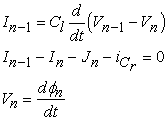(1)

Where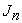is the current through the JJ at cell n. One can derive from the equation Eq. (1)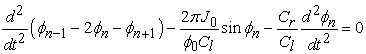(2)

Where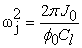is the Josephson plasma frequency and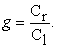Eq. (2) can be written in the form,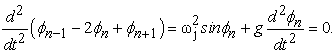(3)

where the parameter are given by (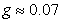,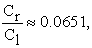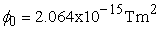)

The right side of Eq. (3) can be approximated with partial derivatives with respect to the distance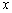from the beginning of the line, assuming that the spacing between two adjacent sections is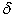with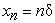. Let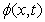be a continuous function of the variables x and t, so that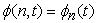and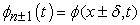.

The magnetic flux at the nodes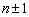can be written using taylor expansion in the form: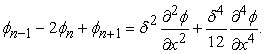(4)

By substituting Eq. (4) in Equation Eq. (3), we obtain the following nonlinear differential equation for the magnetic flux.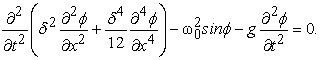(5)

### 3. Derivation of an Exact Solitary Wave Solution

In order to determine analytical solution of the JNLHTL, we apply Riccati method. The first step is to introduce the magnetic flux in the form of travelling wave.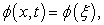where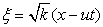,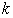and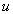are undetermined parameter, and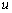is the velocity of propagation.

We can transform equation Eq.(6) to the ordinary differential equation (ODE), and we set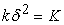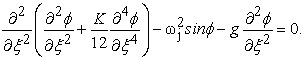(6)

By integrating equation Eq. (6) doubly, and taking into account that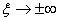the constants are zero, we arrive at the result below,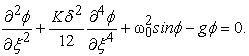(7)

We can consider the small signal, or the phase constant of the JNLH is expressed in the form,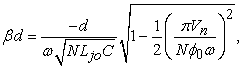(8)

and considering the Taylor’s approximations of order two in this case , the equation takes the following form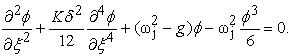(9)

Considering the different calculation steps and the Ricatti resolution mechanism applied in 11, 12, 13.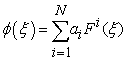(10)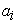is a constant with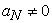to be determined and N positive integer to be determined as well. The function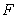expresses the solution of the following generalized Riccati equation: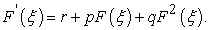(11)

In the case of the ordinary differential equation (ODE) Eq (9), we find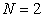, applying to the equation Eq (10), one obtains consequently: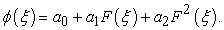(12)

Thus, we succeed in obtaining the one following result, using the computer program MAPLE or MATLAB.

Whereare arbitrary constants non zero,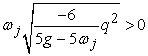because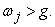.

Inserting the following results in to Eq. (11), and considering the solution of the generalized Riccati equation in 12, we have the exact solutions of Eq. (9) as follows in four cases as presented in 11 and taking into account the remarks made in 13. The solutions are reduced as follows:

i. For the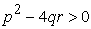and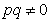or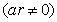the solitons and Solitons solutions of Eq. (9) are: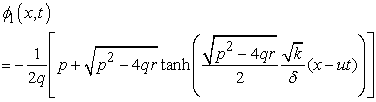(13)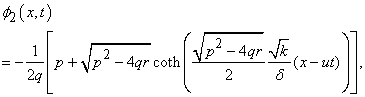(14)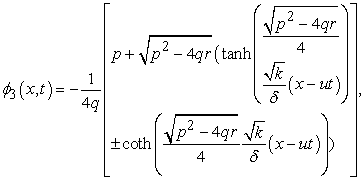(15)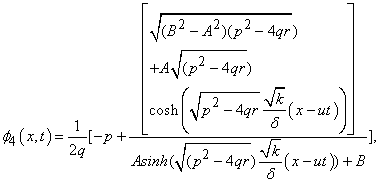(16)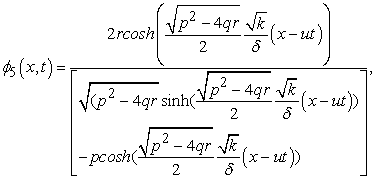(17)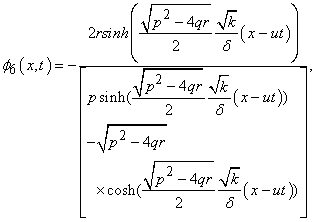(18)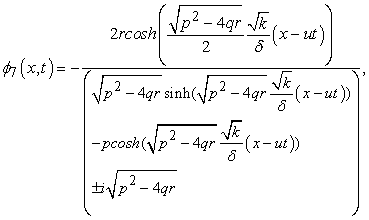(19)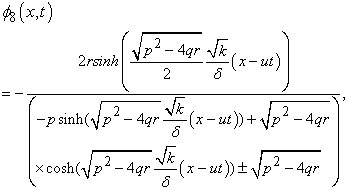(20)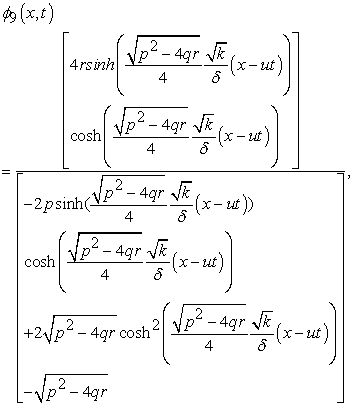(21)

Where A and B are real constants nonzero,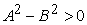.

ii. For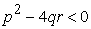andor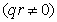, the periodic solutions of the equation Eq (9) are given: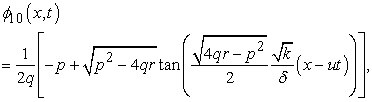(22)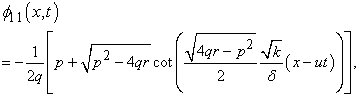(23)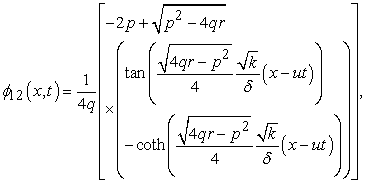(24)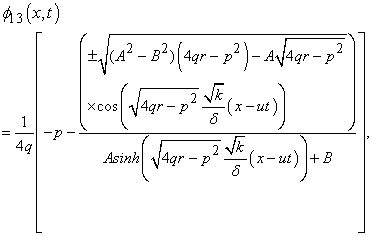(25)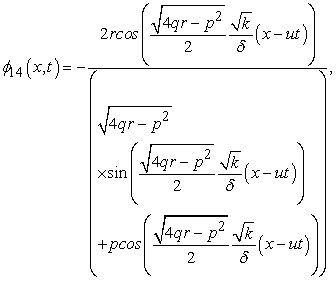(26)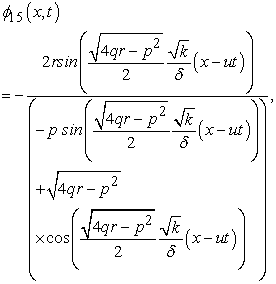(27)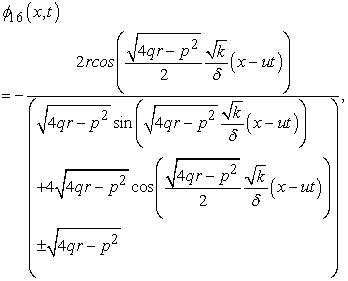(28)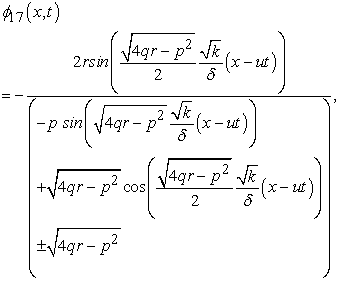(29)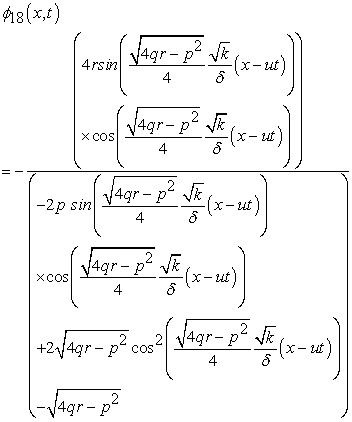(30)

Where A and B are real constant nonzero with.

iii. When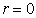and, solitons solutions of the equation Eq(9) are: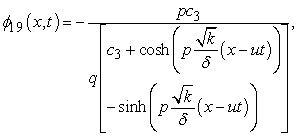(31)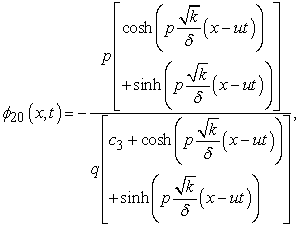(32)

where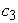is arbitrary constant.

iv. For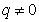and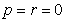, the solitons solutions of Eq. (9) are: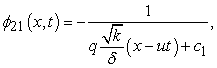(33)

where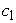is an arbitrary constant

For the results obtained in the table, the nature of the solitons depends on the values of the constants and the soliton solution.

For the results, using the form of the solution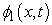, we obtain the following soliton solution: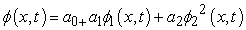(34)

we have types of solitons which follow the curves of Figure 2 and Figure 3. Kink solitons obtained in Figure 2, can be used to generation nonlinear localized modes or discrete breathers Kink and anti-kink collision. In the oder hand, Brigth soliton results from a suitable compensation between nonlinear and dispersive effects of JNLH TL.

• Figure 2. Evolution of kink solitons
• Figure 3. Evolution of Brigth soliton

Using the form of the solution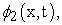we obtain the following soliton solution: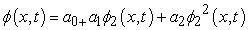(35)

we have types of solitons which follow the curves of Figure 4 and Figure 5.

• Figure 4. The nonlinear evolution behavior of pulse train
• Figure 5. Evolution of nonlinear pulse train

Using the form of the solution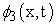, we obtain the following soliton solution, in accordance with the results obtained in 17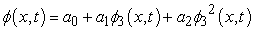(36)

we obtain profile of the dark soliton which follow the curves. This result was obtained in the context of the work presented in the non-linear transmission line in 17.

• Figure 6. Profile of the dark soliton solution expressed via solution of Eq.(13)

We see clearly that the form of the solution depends on certain parameters, and this agrees with ref. 15, 16, 17. The existence of soliton envelopes, kink solitons in the JNLHTL is confirmed.

### 4. Conclusion

In this paper, by means of Ricati method, we have obtained exact solitary wave solutions of the nonlinear equation describing wave propagation in nonlinear Josephson left-handed transmission line. It has been shown that a NL LH TL loaded with Josephson junction supports envelope soliton (dark and bright soliton) as optical fibers in their normal dispersion regime. In addition, one notices the formation and displacement of kink-soliton which is the line feature.

### Acknowledgements

As is a grateful to the Ministry of Higth Education and Ministry of Livestock, Fisheries and Animal Industries of Cameroon for a partial financial support under the program Academic Mobility.

### References

  H. Salehi, R.R. Mansour and A.H. Majedi, Nonlinear Josephson left-handed transmission lines, IET Microw. Antennas Propag., 2007, 1, (1), pp. 69-72. In article View Article  K, Alan M., Introduction to superconducting circuits, New York: Wiley, c1999. In article  A. Barone, G. Paterno, “Physics and applications of the Josephson effect”, 2nd Edition, John Wiley & Sons, 1982. In article View Article  PubMed  McLaughlin DW, CScott A. Phys Rev A 1978;18: 1652. In article View Article  Salehi H, Mansour RR, Majedi AH. IET Microwaves Antennas & Propagation 2007; 1: 69. In article View Article  Collin RE. Field Theory of Guided Waves. Oxford: Oxford University Press; 1991. In article View Article  A. Shahvarpour, S. Gupta, and C. Caloz, J. Appl. Phys. 104, 1245102008. In article  S. Gupta and C. Caloz, IEEE MTT-S Int. Microwaves Symp. Dig. 1,979 2007. In article  A. B. Kozyrev, and D.W. van der Weide, ‘Nonlinear wavepropagation phenomena in left-handed transmission-line media’, IEEE Trans. Microw. Theory Tech., 2005, 53, (1), pp. 238-245. In article View Article  A. Barone, G. Paterno, “Physics and applications of the Josephson effect”, 2nd Edition, John Wiley & Sons, 1982. In article View Article  PubMed  B. H. Malwe, G. Betchewe,·S. Y. Doka, “Travelling wave solutions and soliton solutions for the nonlinear transmission line using the generalized Riccati equation mapping method”, Springer Science+Business Media Dordrecht 2015. In article View Article  Shun-don, Z. “The generalizing Riccati equation map- ping method in non-linear evolution equation: application to (2+1)-dimensional Boiti–Leon–Pempinelle equation. Chaos Solitons Fract”. 37, 1335-1342 (2008). In article View Article  Zheng, C.L. “Comment on the generalizing Riccati equation mapping method in nonlinear evolution equation: application to (2+1)-dimensionalBoiti–Leon–Pempinelle equation. Chaos Solitons Fract”. 39, 1493-1495 (2009). In article View Article  L.Q. English, S.G. Wheeler, Y. Shen b, G.P. Veldes, N. Whitaker, P.G. Kevrekidis, D.J. Frantzeskakis, “Backward-wave propagation and discrete solitons in a left-handed electrical lattice”, Physics Letters A 375 (2011) 1242-1248, (2011). In article View Article  S. Abdoulkary, L.Q. English, A. Mohamadoud, “Envelope solitons in a left-handed nonlinear transmission line with Josephson junction”, Chaos, Solitons and Fractals 85 (2016) 44-50, (2016). In article View Article  Z. Wang, Y. Feng, B. Zhu, J. Zhao, and T. Jiang, “Dark Schrödinger solitons and harmonic generation in left-handed nonlinear transmission line”, Journal of Applied Physics 107, 094907 (2010). In article View Article  S. ABDOULKARY and al, dynamics of solitary pulses in the nonlinear low-pass electrical transmission lines through the auxiliary equation method, J. Mod. Phys. Appl. 2 (2013). In articleThis work is licensed under a Creative Commons Attribution 4.0 International License. To view a copy of this license, visit http://creativecommons.org/licenses/by/4.0/